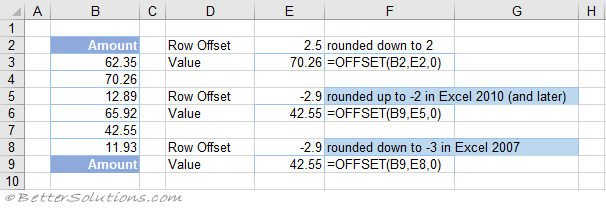# OFFSET - Rounding Bug

The OFFSET function returns the value in a cell which is an offset from another cell.
The behaviour in Excel 2010 (and later) is different to Excel 2007.
If the "rows" argument is not an integer, then the truncating and rounding is different for negative numbers.
If the "cols" argument is not an integer, then the truncating and rounding is different for negative numbers.In Excel 2007 the value returned from the formula in cell "E9" was 65.92
In Excel 2010 (and later) the value returned from the formula in cell "E6" is 42.55